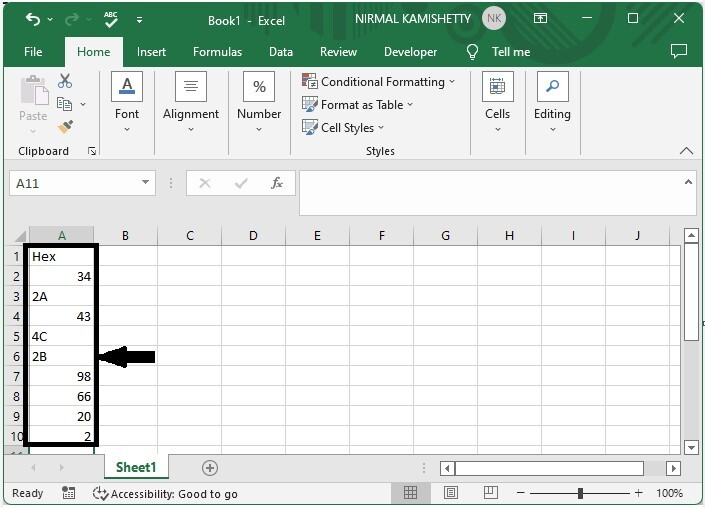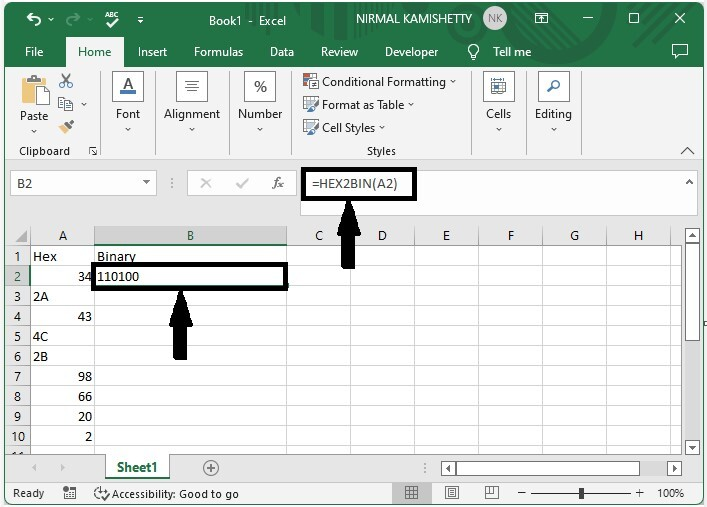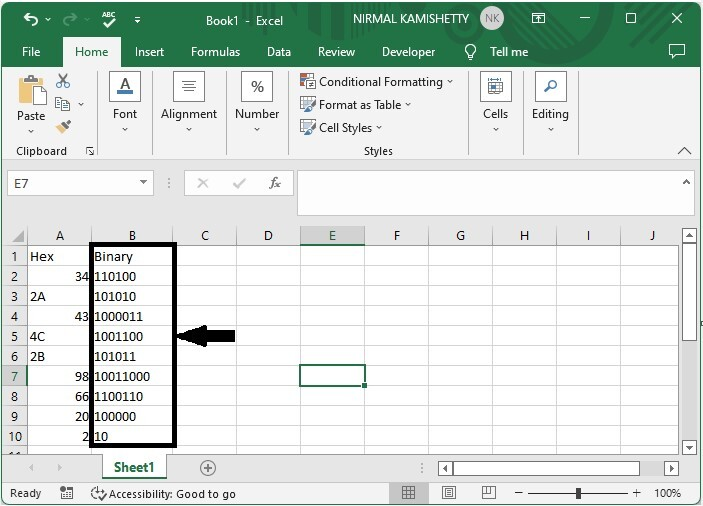# How to Convert Hex to Binary in Excel?

There are typically four different types of numbers: decimal, binary, octal, and hexadecimal. The base values for binary, decimal, octal, and hex numbers are 2, 10, 8, and 16, respectively. Using Excel formulas, we can change a hex number into a binary number. The base values of the hexadecimal number, which has a value of 16, are 0–9 and the letters A–F. The base values for the decimal number, which has a base of 10, are 0 through 9. The base values of the decimal number are 0 and 1, and its base value is 2. The bases for an octal number are 0 through 7. This tutorial will demonstrate how you can change hexadecimal numbers in Excel to binary numbers and vice versa.

## Convert Hex to Binary in Excel

Here, we will first use the formula to get any one of the results, then use the autofill handle to get all the results. Let us look at a simple procedure for converting a hex number to a binary number in Excel using formulas.

### Step 1

Consider an Excel sheet with a list of hexadecimal numbers similar to the image below.To convert the hexadecimal number to a binary number, click on an empty cell, in our case cell B2, and enter the formula =HEX2BIN(A2), then press enter to obtain our first result, as shown in the image below.

Empty cell > formula > enter### Step 2

Now, to get all the values we need, we need to drag them down from the first cell using the auto-fill handle, and our final result will be similar to the below image.We can use the formula =BIN2HEX(A2) to convert a binary number to a hex number .In the formula, A2 is the address of our value in the Excel sheet.

## Conclusion

In this tutorial, we used an example to demonstrate how you can convert hex to binary in Excel.

Updated on: 06-Mar-2023

2K+ Views